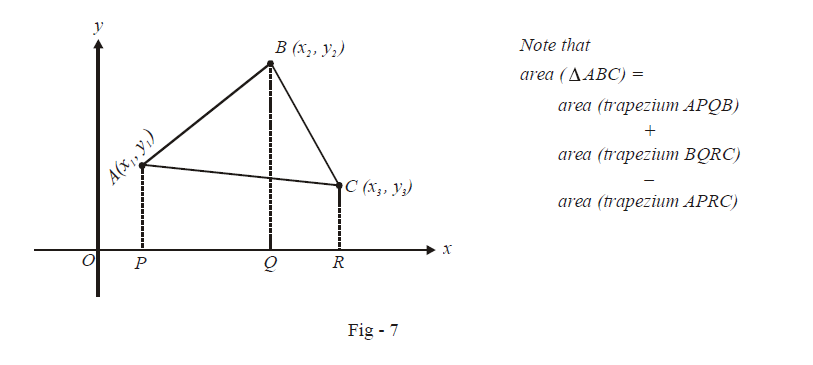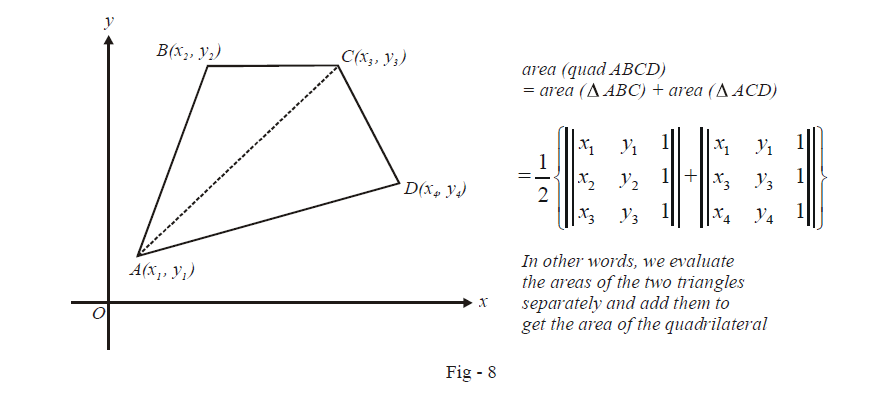# Area of a Triangle Formula

Go back to  'Straight Lines'

$$\textbf{Art 3 :}\qquad\boxed{{\text{Area of a triangle}}}$$

Suppose we are given three points in the co-ordinate plane :  $$A\left( {{x}_{1}},\,\,{{y}_{1}} \right),\,\,B\left( {{x}_{2}},\,\,{{y}_{2}} \right)\,\,\text{and}\,\,C\left( {{x}_{3}},\,\,{{y}_{3}} \right).$$ We intend to find the area of $$\Delta \,ABC$$ in terms of the given co-ordinates. How to evaluate this area is described in the figure below:Observe how the area of  $$\Delta \,ABC$$ has been written in terms of the area of three trapeziums.

From plane geometry, the area of a trapezium is \begin{align}\frac{1}{2}\end{align}\times \left( \text{sum of bases} \right)\times \text{height}\text{.} Thus,

\begin{align} & \text{area}\left( \text{trap}.APQB \right)=\frac{1}{2}\times \left( AP+BQ \right)\times PQ \\ &\qquad\qquad\qquad\qquad =\frac{1}{2}\left( {{y}_{1}}+{{y}_{2}} \right)\left( {{x}_{2}}-{{x}_{1}} \right)\qquad \qquad ...\text{ }\left( 1 \right) \end{align}

Similarly,

\begin{align} & \text{area}\left( \text{trap}.\,BQRC \right)=\frac{1}{2}\times \left( BQ+CR \right)\times QR \\ & \qquad\qquad\qquad\qquad\;=\frac{1}{2}\left( {{y}_{2}}+{{y}_{3}} \right)\left( {{x}_{3}}-{{x}_{2}} \right)\qquad \qquad ...\text{ }\left( 2 \right) \\ & \text{area}\left( \text{trap}.\,APRC \right)=\frac{1}{2}\times \left( AP+CR \right)\times PR \\ &\qquad\qquad\qquad\qquad\; =\frac{1}{2}\left( {{y}_{1}}+{{y}_{3}} \right)\left( {{x}_{3}}-{{x}_{1}} \right)\qquad \qquad ...\text{ }\left( 3 \right) \end{align}

From (1), (2) and (3), we have, upon simplification,

\begin{align}& \text{area}\left( \Delta \,ABC \right)=\frac{1}{2}\left( {{x}_{2}}{{y}_{1}}-{{x}_{1}}{{y}_{2}}+{{x}_{3}}{{y}_{2}}-{{x}_{2}}{{y}_{3}}+{{x}_{1}}{{y}_{3}}-{{x}_{3}}{{y}_{1}} \right) \\ &\qquad\qquad\qquad =\left| \frac{1}{2}\left\{ {{x}_{1}}\left( {{y}_{2}}-{{y}_{3}} \right)+{{x}_{2}}\left( {{y}_{3}}-{{y}_{1}} \right)+{{x}_{3}}\left( {{y}_{1}}-{{y}_{2}} \right) \right\} \right| \end{align}

We used the mod sign in the last expression because area is by definition positive.

We can express the area obtained in determinant form very concisely:

$\Delta =\text{area}\left( \Delta \,ABC \right)=\left| \frac{1}{2}\left| \begin{matrix} {{x}_{1}} & {{y}_{1}} & 1 \\ {{x}_{2}} & {{y}_{2}} & 1 \\ {{x}_{3}} & {{y}_{3}} & 1 \\ \end{matrix} \right| \right|$

As a consequence of this result, we can now easily find the area of an arbitrary quadrilateral ABCD as describe in the figure below:We can generalise this method easily to find the area of any polygon as a sum of the areas of the constituent triangles.

Download SOLVED Practice Questions of Area of a Triangle Formula for FREE
Straight Lines
grade 11 | Answers Set 2
Straight Lines
grade 11 | Questions Set 1
Straight Lines
grade 11 | Answers Set 1
Straight Lines
grade 11 | Questions Set 2